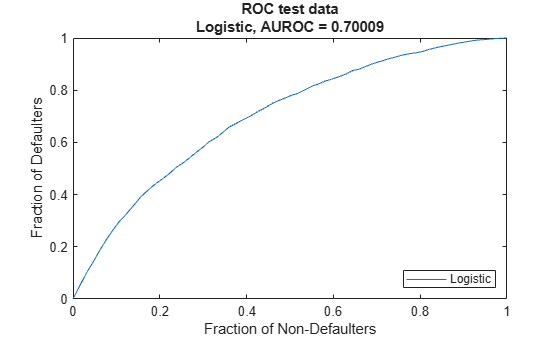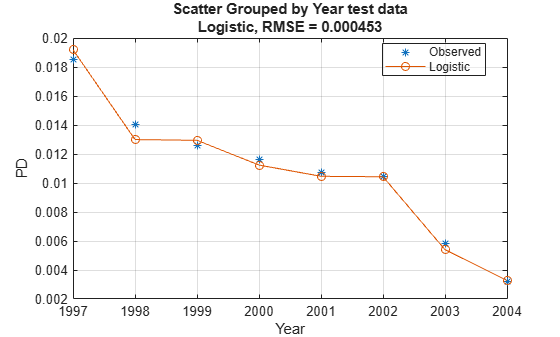Create specified lifetime PD model object type

## Syntax

``pdModel = fitLifetimePDModel(data,ModelType)``
``pdModel = fitLifetimePDModel(___,Name,Value)``

## Description

example

````pdModel = fitLifetimePDModel(data,ModelType)` creates a lifetime probability of default (PD) model object specified by `data` and `ModelType`. `fitLifetimePDModel` takes in credit data in panel data form and fits a lifetime PD model. `ModelType` is supported for `Logistic`, `Probit`, or `Cox`.```

example

````pdModel = fitLifetimePDModel(___,Name,Value)` specifies options using one or more name-value pair arguments in addition to the input arguments in the previous syntax. The available optional name-value pair arguments depend on the specified `ModelType`. ```

## Examples

collapse all

This example shows how to use `fitLifetimePDModel` to create a `Logistic` model using credit and macroeconomic data.

```load RetailCreditPanelData.mat disp(head(data))```
``` ID ScoreGroup YOB Default Year __ __________ ___ _______ ____ 1 Low Risk 1 0 1997 1 Low Risk 2 0 1998 1 Low Risk 3 0 1999 1 Low Risk 4 0 2000 1 Low Risk 5 0 2001 1 Low Risk 6 0 2002 1 Low Risk 7 0 2003 1 Low Risk 8 0 2004 ```
`disp(head(dataMacro))`
``` Year GDP Market ____ _____ ______ 1997 2.72 7.61 1998 3.57 26.24 1999 2.86 18.1 2000 2.43 3.19 2001 1.26 -10.51 2002 -0.59 -22.95 2003 0.63 2.78 2004 1.85 9.48 ```

Join the two data components into a single data set.

```data = join(data,dataMacro); disp(head(data))```
``` ID ScoreGroup YOB Default Year GDP Market __ __________ ___ _______ ____ _____ ______ 1 Low Risk 1 0 1997 2.72 7.61 1 Low Risk 2 0 1998 3.57 26.24 1 Low Risk 3 0 1999 2.86 18.1 1 Low Risk 4 0 2000 2.43 3.19 1 Low Risk 5 0 2001 1.26 -10.51 1 Low Risk 6 0 2002 -0.59 -22.95 1 Low Risk 7 0 2003 0.63 2.78 1 Low Risk 8 0 2004 1.85 9.48 ```

Partition Data

Separate the data into training and test partitions.

```nIDs = max(data.ID); uniqueIDs = unique(data.ID); rng('default'); % for reproducibility c = cvpartition(nIDs,'HoldOut',0.4); TrainIDInd = training(c); TestIDInd = test(c); TrainDataInd = ismember(data.ID,uniqueIDs(TrainIDInd)); TestDataInd = ismember(data.ID,uniqueIDs(TestIDInd));```

Create `Logistic` Lifetime PD Model

Use `fitLifetimePDModel` to create a `Logistic` model using the training data.

```pdModel = fitLifetimePDModel(data(TrainDataInd,:),"Logistic",... 'AgeVar','YOB',... 'IDVar','ID',... 'LoanVars','ScoreGroup',... 'MacroVars',{'GDP','Market'},... 'ResponseVar','Default'); disp(pdModel)```
``` Logistic with properties: ModelID: "Logistic" Description: "" Model: [1x1 classreg.regr.CompactGeneralizedLinearModel] IDVar: "ID" AgeVar: "YOB" LoanVars: "ScoreGroup" MacroVars: ["GDP" "Market"] ResponseVar: "Default" ```

Display the underlying model.

`disp(pdModel.Model)`
```Compact generalized linear regression model: logit(Default) ~ 1 + ScoreGroup + YOB + GDP + Market Distribution = Binomial Estimated Coefficients: Estimate SE tStat pValue __________ _________ _______ ___________ (Intercept) -2.7422 0.10136 -27.054 3.408e-161 ScoreGroup_Medium Risk -0.68968 0.037286 -18.497 2.1894e-76 ScoreGroup_Low Risk -1.2587 0.045451 -27.693 8.4736e-169 YOB -0.30894 0.013587 -22.738 1.8738e-114 GDP -0.11111 0.039673 -2.8006 0.0051008 Market -0.0083659 0.0028358 -2.9502 0.0031761 388097 observations, 388091 error degrees of freedom Dispersion: 1 Chi^2-statistic vs. constant model: 1.85e+03, p-value = 0 ```

Use the `predict` function to predict conditional PD values. The prediction is a row-by-row prediction.

```dataCustomer1 = data(1:8,:); CondPD = predict(pdModel,dataCustomer1)```
```CondPD = 8×1 0.0092 0.0053 0.0045 0.0039 0.0037 0.0037 0.0019 0.0012 ```

Use `predictLifetime` to predict the lifetime cumulative PD values (computing marginal and survival PD values is also supported). The `predictLifetime` function uses the ID variable (see the `'IDVar'` property for the `Logistic` object) to transform conditional PDs to cumulative PDs for each ID.

`LifetimePD = predictLifetime(pdModel,dataCustomer1)`
```LifetimePD = 8×1 0.0092 0.0145 0.0189 0.0228 0.0264 0.0300 0.0319 0.0330 ```

Validate Model

Use `modelDiscrimination` to measure the ranking of customers by PD.

```DiscMeasure = modelDiscrimination(pdModel,data(TestDataInd,:),'DataID','test data'); disp(DiscMeasure)```
``` AUROC _______ Logistic, test data 0.70009 ```

Use `modelDiscriminationPlot` to visualize the ROC curve.

`modelDiscriminationPlot(pdModel,data(TestDataInd,:),'DataID','test data');`Use `modelAccuracy` to measure the accuracy of the predicted PD values, also known as model calibration. The `modelAccuracy` function requires a grouping variable and compares the accuracy of the observed default rate in the group with the average predicted PD for the group. For example, you can group by calendar year using the `'Year'` variable.

```AccMeasure = modelAccuracy(pdModel,data(TestDataInd,:),'Year','DataID','test data'); disp(AccMeasure)```
``` RMSE ________ Logistic, grouped by Year, test data 0.000453 ```

Use `modelAccuracyPlot` to visualize the observed default rates compared to the predicted probabilities of default (PD).

`modelAccuracyPlot(pdModel,data(TestDataInd,:),'Year','DataID','test data');`## Input Arguments

collapse all

Data, specified as a table, in panel data form. The `data` must contain an ID column. The response variable must be a binary variable with the value `0` or `1`, with `1` indicating default.

Data Types: `table`

Type of PD model, specified as a scalar string or character vector. Use one of following values: `'Logistic'`, `'Probit'`, or `'Cox'`.

Data Types: `string` | `char`

### Name-Value Arguments

Specify optional comma-separated pairs of `Name,Value` arguments. `Name` is the argument name and `Value` is the corresponding value. `Name` must appear inside quotes. You can specify several name and value pair arguments in any order as `Name1,Value1,...,NameN,ValueN`.

Example: ```pdModel = fitLifetimePDModel(data(TrainDataInd,:),ModelType,'AgeVar',"YOB",'IDVar',"ID",'LoanVars',"ScoreGroup",'MacroVars',{'GDP','Market'},'ResponseVar',"Default")```

The available name-value pair arguments depend on the value you specify for `ModelType`.

Name-Value Pair Arguments for Model Objects

## Output Arguments

collapse all

Probability of default model, returned as a `pdModel` object. Supported classes are `Logistic`, `Probit`, or `Cox`.

Independently published

 Baesens, Bart, Daniel Roesch, and Harald Scheule. Credit Risk Analytics: Measurement Techniques, Applications, and Examples in SAS. Wiley, 2016.

 Bellini, Tiziano. IFRS 9 and CECL Credit Risk Modelling and Validation: A Practical Guide with Examples Worked in R and SAS. San Diego, CA: Elsevier, 2019.

 Breeden, Joseph. Living with CECL: The Modeling Dictionary. Santa Fe, NM: Prescient Models LLC, 2018.

 Roesch, Daniel and Harald Scheule. Deep Credit Risk: Machine Learning with Python. Independently published, 2020.